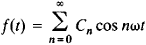# Oscillation Spectrum

## Spectrum, Oscillation

a set of simple harmonic oscillations into which a given complex oscillatory motion can be resolved. Mathematically, such motion can be represented by a periodic but nonharmonic function f(t) having the frequency ω. This function can be expanded into a spectrum—that is, it can be represented as a series of harmonic functionswith frequencies nω, which are whole multiples of a fundamental frequency ω. Here, Cn is the amplitude of the nth harmonic function, t is time, and n is the number of the harmonic.

The more a complex oscillation differs from the harmonic type, the richer is its spectrum, the more overtones there are contained in the expansion, and the larger are the amplitudes of the overtones. In the general case, the spectrum of a periodic oscillation contains an infinite series of overtones, whose amplitudes decrease as the number of the overtone increases. Since the amplitudes decrease fairly rapidly, in practice only a finite number of overtones need be taken into consideration.

Processes that are not strictly periodic or are nonperiodic can be represented as (1) a sum of harmonic components whose frequencies are not multiples or (2) a sum (integral) of an infinite number of components whose frequencies are arbitrarily close to each other. In the second case, the spectrum is said to be continuous.

Depending on the nature of the oscillatory process, optical, electrical, and mechanical spectra are distinguished. A sound spectrum is an example of a mechanical spectrum.

Mentioned in ?
References in periodicals archive ?
It is supposed that particularly the 1st and 2nd eigenfrequencies of the drive system (approximately 14 Hz and 29 Hz) as well as higher frequency peaks will be recognizable in the oscillation spectrum.
In other words one may expect some effects with respect to Earth eigen oscillation spectrum, which is related to the Earth's inner core interior.
These fluctuations are quite rapid and may result in the very wide oscillation spectrum shown in Figure 5, even with a nonvarying load.

Site: Follow: Share:
Open / Close